[可分离核（退化核）]  若核K(x,x )可分解为如下的形式：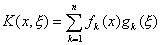[具有可分离核的第二类Fr方程解法]  具有可分离核的第二类Fr方程

`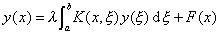(1)`

`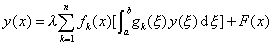(2)`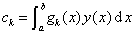(k=1,2,L,n)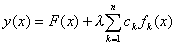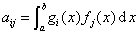i=1,2,L,n ,  j=1,2,L,n

c1,c2,L,cn满足方程组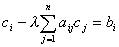i=1,2,L,n

`（3）`

I-lAc=b

D=detI-l A¹0

解积分方程

`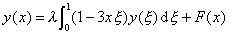(1)`

可把这个方程改写为

`                       y(x)=l(c1-3c2x)+F(x)                                    (2)`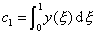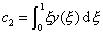`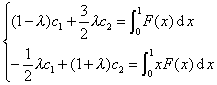(3)`λ=2，则方程组(3)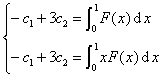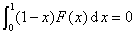λ=-2，则方程组(3)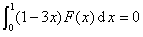先考虑齐次方程（即F(x)=0）的情形。若l ¹±2，则唯一解是平凡解y(x)=0

λ=2时，代数方程组只给出一个条件c1=3c2。这时，解是

y(x)=c1(1-x)

λ=-2时，解是

y(x)=c2(1-3x)

y(x)=F(x)+c3(1-x)+c4(1-3x)

λ=2时，积分方程(1)没有解，除非在区间[0,1]F(x)正交于λ=2所对应的特征函数1-x*,即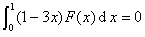* 在下一段会看到，这个情形是原积分方程中核K(x,ξ)=1-3xξ  的对称性的一个推论。